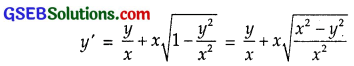GSEB Solutions Class 12 Maths Chapter 9 Differential Equations Ex 9.2

Gujarat Board GSEB Textbook Solutions Class 12 Maths Chapter 9 Differential Equations Ex 9.2 Textbook Questions and Answers.

Gujarat Board Textbook Solutions Class 12 Maths Chapter 9 Differential Equations Ex 9.2

In each of the questions 1 to 10, verify that the given function (explicit or implicit) is a solution of the corresponding differential equation:
Question 1.
y = ex + 1 : y” – y’ = 0
Solution:
y = ex + 1
Differentiating w.r.t. x,
y’ = ex + 0 ……………. (1)
Again differentiating,
y” = ex ……………….. (2)
Subtracting (1) from (2),
y” – y’ = ex – ex = 0.
∴ Required differential equation is y” – y’ = 0.Question 2.
y = x2 + 2x + C : y’ – 2x – 2 = 0
Solution:
y = x2 + 2x + C
Differentiating w.r.t.x,
y’ = 2x + 2
⇒ y’ – 2x – 2 = 0
∴ Required differential equation is y’ – 2x – 2 = 0.

Question 3.
y = cos x + C : y’ + sin x = 0
Solution:
y = cos x + C
Differentiating w.r.t. x,
y’ = – sin x or y + sin x = 0
∴ Required differential equation is y’ + sin x = 0

Question 4.
y = $$\sqrt{1+x^{2}}$$ : y’ = $$\frac{x y}{\sqrt{1+x^{2}}}$$
Solution:Hence, the required differential equation is y’ = $$\frac{x y}{1+x^{2}}$$.Question 5.
y = A x : xy’ = y, (x ≠ 0)
Solution:
y = Ax ………….. (1)
Differentiating w.r.t. x,
y’ = A …………….. (2)
Eliminating A from equations (1) and (2),
y = y’x
∴ The required differential equation is xy’ = y. (x ≠ 0)

Question 6.
y = x sin x : xy’ = y + x $$\sqrt{x^{2}-y^{2}}$$ (x ≠ 0 and x > y or x < – y)
Solution:
y = x sin x …………….. (1)
Differentiating w.r.t. x,
y’ = 1.sin x + x cos x
= sin x + x cos x [∵ sin2x + cos2x = 1] ……………… (2)
From (1), sin x = $$\frac{y}{x}$$
Putting this value sin x in (2), we getMultiplying by x, we get
xy’ = y + x$$\sqrt{x^{2}-y^{2}}$$
Which is the required differential equation.Question 7.
xy = log y + C : y’ = $$\frac{y^{2}}{1-x y}$$ (xy ≠ 1)
Solution:
xy = log x + C
Differentiating w.r.t. x,
1.y + xy’ = $$\frac{1}{y}$$y’
or y2 + xy y’ = y’
or y2 = y’ – xy y’ = y'(1 – xy)
y’ = $$\frac{y^{2}}{1-x y}$$.
which is the required differential equation.

Question 8.
y – cos y = x : (y sin y + cos y + x)y’ = y
Solution:
y – cos y = x ……………… (1)
Differentiating w.r.t. x,
(1 + sin y)y’ = x
Multiplying both sides by y,
(y + sin y)y’ = y ………………… (2)
From (1), y = x + cos y.
Putting it in (2), we get
(x + cos y + y sin y)y’ = y
or (y sin y + cos y + x)y’ = y,
which is the required differential equation.Question 9.
x + y = tan-1y : y2y’ + y2 + 1 = 0
Solution:
x + y = tan-1y
Differentiating w.r.t. x,
1 + y’ = $$\frac{y^{\prime}}{1+y^{2}}$$
(1 + y2) + y'(1 + y2) = y’
or 1 + y2 + y’ (1 + y2 – 1) = 0
or y2y’ + y2 + 1 = 0,
which is the required differential equation.

Question 10.
y = $$\sqrt{a^{2}-x^{2}}$$ x ∈ (- a, a) : x + y $$\frac{dy}{dx}$$ = 0, y ≠ 0
Solution:
y = $$\sqrt{a^{2}-x^{2}}$$
Squaring both sides, y2 = a2 – x2
or x2 + y2 = a2
Differentiating w.r.t. x,
2x + 2yy’ = 0
Dividing by 2, x + y $$\frac{dy}{dx}$$ = 0,
which is the required differential equation.Choose the correct answers in the following questions 11 and 12:

Question 11.
The number of arbitarary constants in the general solution of a differential equation of fourth order is
(A) 0
(B) 2
(C) 3
(D) 4
Solution:
Number of constants in general solution = order of the differential equation.
The differential equation is of fourth order.
∴ Number of arbitarary constants = 4
∴ Part (D) is the correct answer.Question 12.
The number of arbitarary constants in the particular solution of a differential equation of third order is
(A) 3
(B) 2
(C) 1
(D) 0
Solution:
In a particular solution of a differential equation, there is no arbitarary constant.
∴ Part (D) is the correct answer.